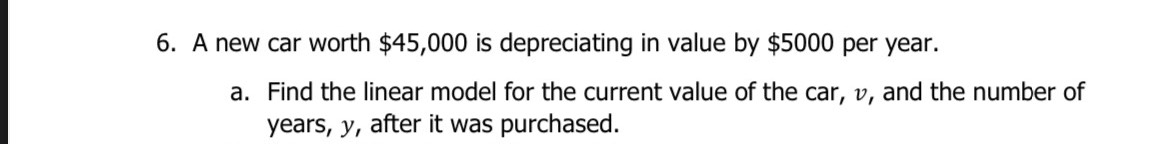### ¿Todavía tienes preguntas de matemáticas?

Pregunte a nuestros tutores expertos
Algebra
Pregunta6. A new car worth $$\ 45,000$$ is depreciating in value by $$\ 5000$$ per year. a. Find the linear model for the current value of the car, $$v$$ , and the number of years, $$y$$ , after it was purchased.